# Geometric Shapes Worksheets

#### What Are the Common Geometric Shapes?

Geometry is a branch of mathematics that one can relate to the day-to-day life. It is the study of shapes, positions, angles, and dimensions. Wherever one looks, geometry concepts are applied everywhere. To excel in this mathematical branch, one needs to be well aware of the basic shapes. The principal geometric shapes include; Circles - It is a shape that can be made by tracing a curve that has the same distance from a point, known as the center. The total boundary of this shape is called the circumference. Triangle - It is a shape that is made of three straight lines. It has three sides and three angles. There are a variety of different triangles, and these include equilateral, scalene, isosceles, right triangle, obtuse triangle, and acute triangle. Rectangle - It is a shape made of four lines that make up four sides. The opposite sides of this shape are equal, and all four angles measure 90°. Square - It is made of four straight lines and has four sides. All four sides are equal, and all four angles measure 90°. Rhombus - It is shape made of four straight lines, which make up four sides of equal length. Any of all four angles measures 90°. Trapezoid - It is a shape made of four straight lines that make four sides. Two opposite sides are parallel, but the other two are not.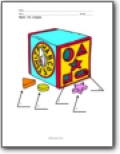###### Name Shapes

You are given a picture of a toy and asked to name all the shapes that you can. There are even more embedded in the picture than we point out for you.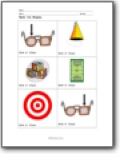###### Everyday Objects

Name all of the shapes that are found within everyday objects.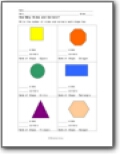###### How Many Sides and Corners?

Write the number of sides and corners each shape has.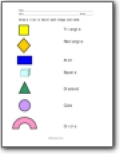###### Word Match

Draw a line to match each shape and name.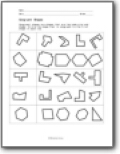###### Congruent Shapes

Congruent shapes are shapes that are the same size and shape. Circle the shape that is congruent to the first shape in each row.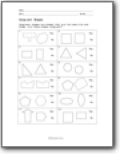###### Congruent?

Congruent shapes are shapes that are the same size and shape. Are these shapes congruent?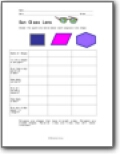###### Sun Glass Lens

Answer the questions below about each sunglass lens shape.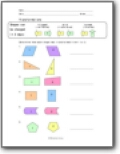###### Transformations

Determine how each shape was transformed from 1 to 2.